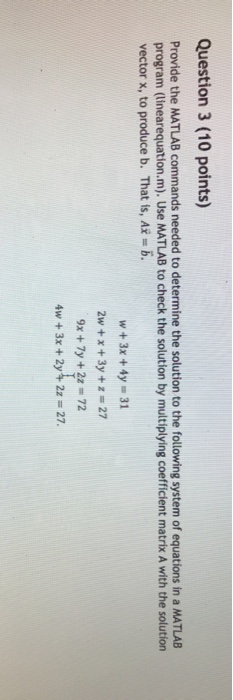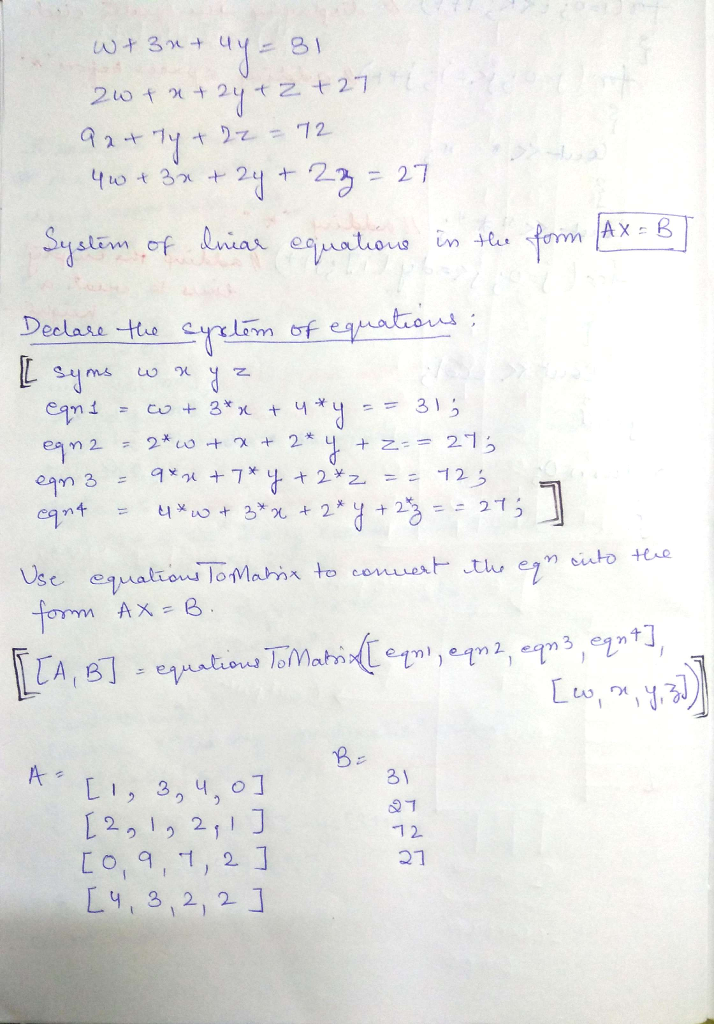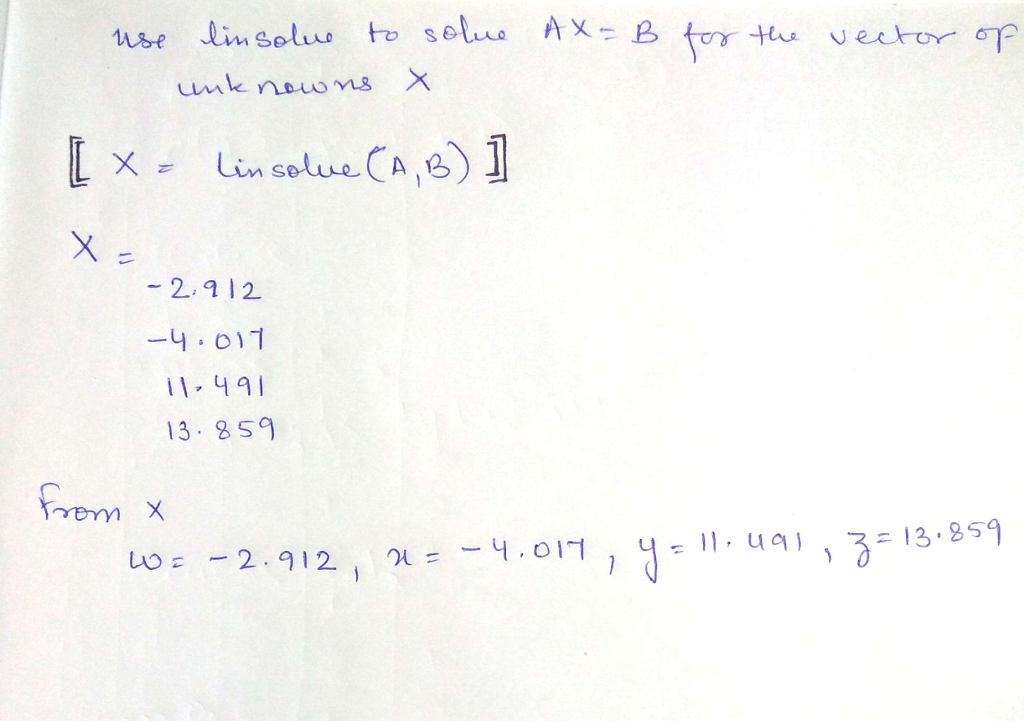# Homework Solution: Provide the MATLAB commands needed to determine the solution to the following system of e…Provide the MATLAB commands needed to determine the solution to the following system of equations in a MATLAB program (linearequation.m). Use MATLAB to check the solution by multiplying coefficient matrix A with the solution vector x, to produce b. That is, Ax = b. w + 3x + 4y = 31 2w + x + 3y + z = 27 9x + 7y + 2z = 72 4w + 3x + 2y + 2z = 27.